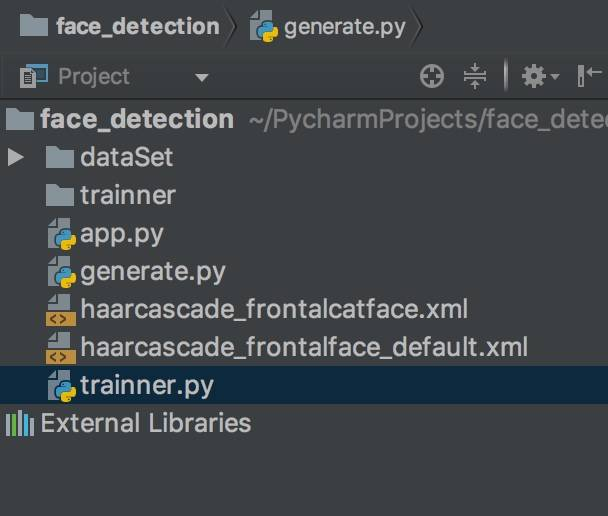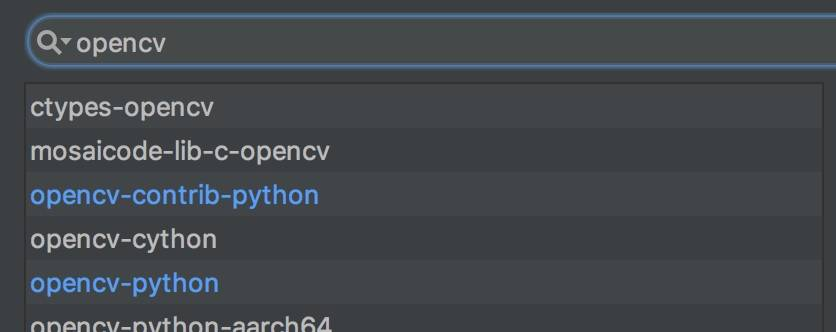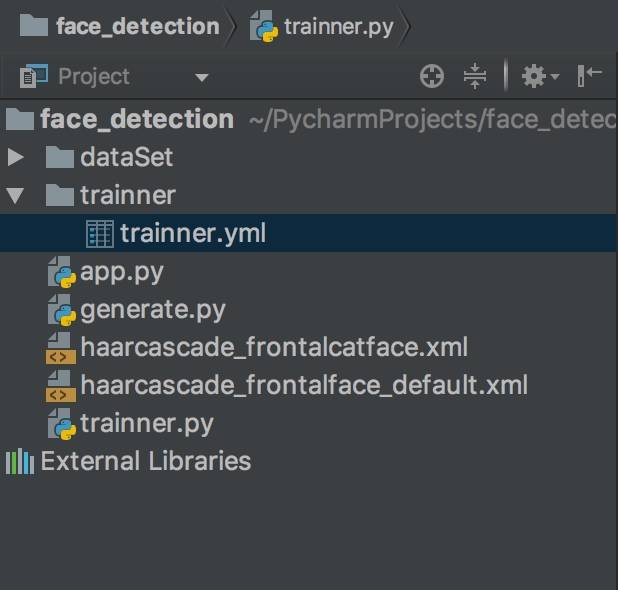# Python-OpenCV训练一个人脸识别器

## 准备``````pip install pillow
``````

## 开始Coding

### 导入库

1. 导入opencv库
2. 导入os库，用于方法文件
3. 导入numpy库，用于计算
4. 导入pillow库，用于处理图像

``````import cv2
import os
import numpy as np
from PIL import Image
``````

``````recognizer = cv2.face.LBPHFaceRecognizer_create()
# 有可能是 recognizer = cv2.createLBPHFaceRecognizer()
``````

``````pip install opencv-contrib-python
``````### 载入训练数据

``````def get_images_and_labels(path):
``````

1. 从数据集文件夹中载入训练图片
2. 获取到人脸和id
3. 整理成list并返回

``````image_paths=[os.path.join(path, f) for f in os.listdir(path)]
``````

``````face_samples=[]
ids=[]
``````

``````    for image_path in image_paths:
image = Image.open(image_path).convert('L')
image_np = np.array(image, 'uint8')
image_id = int(os.path.split(image_path)[-1].split("."))
faces = detector.detectMultiScale(image_np)
for (x, y, w, h) in faces:
face_samples.append(image_np[y:y + h, x:x + w])
ids.append(image_id)
``````

### 训练

``````faces, Ids = get_images_and_labels('dataSet')
recognizer.train(faces, np.array(Ids))
recognizer.save('trainner/trainner.yml')
``````### 完整代码

``````import cv2
import os
import numpy as np
from PIL import Image

# recognizer = cv2.createLBPHFaceRecognizer()
recognizer = cv2.face.LBPHFaceRecognizer_create()

def get_images_and_labels(path):
image_paths = [os.path.join(path, f) for f in os.listdir(path)]
face_samples = []
ids = []

for image_path in image_paths:
image = Image.open(image_path).convert('L')
image_np = np.array(image, 'uint8')
if os.path.split(image_path)[-1].split(".")[-1] != 'jpg':
continue
image_id = int(os.path.split(image_path)[-1].split("."))
faces = detector.detectMultiScale(image_np)
for (x, y, w, h) in faces:
face_samples.append(image_np[y:y + h, x:x + w])
ids.append(image_id)

return face_samples, ids

faces, Ids = get_images_and_labels('dataSet')
recognizer.train(faces, np.array(Ids))
recognizer.save('trainner/trainner.yml')
``````#### weixin_王玮_1 · 06/24/2018 at 00:49#### clarence · 10/30/2018 at 20:18

python3 没有 createLBPHFaceRecognizer 这个模块了,

> pip install opencv-contrib-python

recognizer = cv2.face_LBPHFaceRecognizer.create()#### 坏坏 · 12/02/2018 at 18:39#### 肉肉 · 03/28/2019 at 22:50

2. 在dataSet里有100张我的照片，然而识别的时候别人的脸和我的照片之间的conf总是出奇的高（95之类的），想问一下为什么？#### 煎鱼 · 03/28/2019 at 23:02

1. app和generate是之前生成训练数据用到的文件，详情可以翻之前的文章，https://www.mkshell.com/face-detection-using-opencv-in-python/，https://www.mkshell.com/face-recognition-dataset-generator/。 有trainer和yml，就证明你已经训练过数据了，有模型就可以直接去拿去识别了：https://www.mkshell.com/python-opencv-face-recognition/。
2. 识别时，conf越高，代表识别的置信率越高，就越可信（当然，也有可能是误判）。
3. 最后，希望你不只是学习这么浅的模型，可以感受下CNN那些更深的模型，识别率会高很多。#### long · 07/30/2019 at 16:26#### Benja · 03/15/2020 at 15:42

mac用户一定要记得删除dataset下的隐藏文件.Ds_Store 不然疯狂报错#### badage · 06/20/2020 at 11:08

py版本为3.7但是recognizer = cv2.face.LBPHFaceRecognizer_create()我的cv2找不到face，求解·